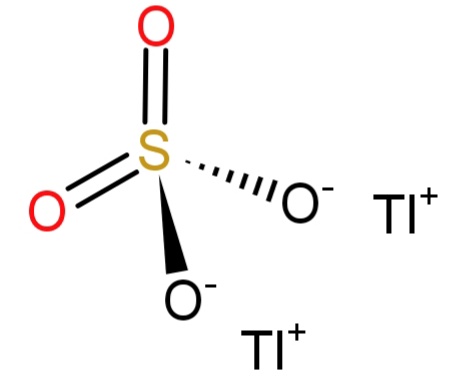QuestionAnswers

# Formula for the following compound Thallium (I) sulphate is $T{l_2}S{O_4}$ .A.TrueB.FalseVerified
129.9k+ views
Hint:
Thallium has 3 valence electrons (2 in 6s and 1 in 6p) and exhibits mostly (+1) oxidation state while sulphate ($S{O_4}^{ - 2}$) exhibits (-2) oxidation state.

-Thallium (Tl) has an atomic number of 81 and belongs to the Boron (B) group. It belongs to the 13th group and 6th period. Hence, its electronic configuration is: $\left[ {Xe} \right]4{f^{14}}5{d^{10}}6{s^2}6{p^1}$.
-Thallium sulphate is colourless, tasteless, odourless and highly toxic (hence used in insecticides, rodenticides and rat poisons. It gains its toxicity due to $T{l^{ + 1}}$ ion.
-Since the question says the name of the formula to be: Thallium (I) sulphate. The number (I) here shows the oxidation state of thallium atom, which is 1. And we all know that the sulphate has the molecular formula of $S{O_4}^{ - 2}$ and has an oxidation state of (-2). To satisfy or neutralise the (-2) charge of sulphate we will need a (+2) charge, but thallium has (+1) charge. So, we will require 2 atoms of thallium. Hence the molecular formula would be: $T{l_2}S{O_4}$.